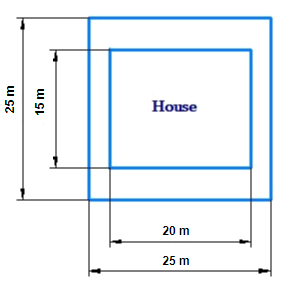# Ex.11.1 Q2 Mensuration Solutions - NCERT Maths Class 8

Go back to  'Ex.11.1'

## Question

Mrs. Kaushik has a square plot with the measurement as shown in the figure. She wants to construct a house in the middle of the plot. A garden is developed around the house. Find the total cost of developing a garden around the house at the rate of $$55$$ per$${{\,\rm{m}}^2}$$.Video Solution
Mensuration
Ex 11.1 | Question 2

## Text Solution

What is Known?

The cost of developing a garden around the house at the rate of per $$\rm m^2$$ and also dimensions of the house and square plot.

What is unknown?

Total cost of developing of garden around the house.

Reasoning:

The plot is square from outside while the area of the house to be constructed is rectangular in the middle of the plot. From the diagram, we can see that the area of the garden is the difference between area of the square plot and the area of the house.

Steps:

Area of the square plot

\begin{align} & = {\rm{side}} \times {\rm{side}} \\ &= 25{\rm{m}} \times 25{\rm{m}} \\ &= 625{{\rm{m}}^2} \end{align}

Area of the house

\begin{align}&=\text{length}\times \text{breadth}\\ & =15\text{m}\times 20\text{m}\\ &=300 \; {{\text{m}}^{2}} \end{align}

Area of the garden to be developed $$=$$ (Area of the square plot ) $$-$$ (Area of the house)

\begin{align} &= 625{{\rm{m}}^2} - 300{{\rm{m}}^2}\\ &= 325{{\rm{m}}^2}\end{align}

Hence Area of the garden to be developed

$$= 325 \, \rm m^2$$

The cost of developing garden around the house

$$= {\rm{Rs}}.55\,\rm\,per\,{m^2}$$

$$\therefore$$ Total cost of developing a garden of area $$325\,{{\rm{m}}^2}$$

\begin{align} &= {\rm{Rs}}.325 \times 55 \\ &= {\rm{Rs}}.17,875\end{align}

Learn from the best math teachers and top your exams

• Live one on one classroom and doubt clearing
• Practice worksheets in and after class for conceptual clarity
• Personalized curriculum to keep up with school